#### The homogeneous transformation matrix

The transformation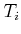, for eachsuch that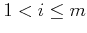, is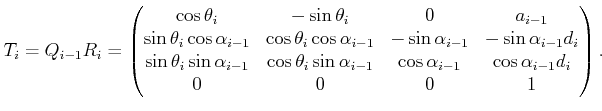(3.56)

This can be considered as the 3D counterpart to the 2D transformation matrix, (3.52). The following four operations are performed in succession:
1. Translate by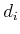along the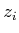-axis.
2. Rotate counterclockwise by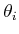about the-axis.
3. Translate by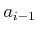along the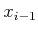-axis.
4. Rotate counterclockwise by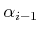about the-axis.

As in the 2D case, the first matrix,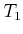, is special. To represent any position and orientation of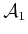, it could be defined as a general rigid-body homogeneous transformation matrix, (3.50). If the first body is only capable of rotation via a revolute joint, then a simple convention is usually followed. Let the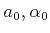parameters ofbe assigned as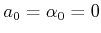(there is no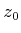-axis). This implies that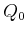from (3.55) is the identity matrix, which makes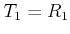.

The transformationfor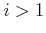gives the relationship between the body frame of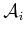and the body frame of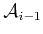. The position of a point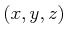on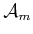is given by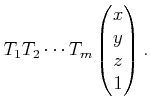(3.57)

For each revolute joint,is treated as the only variable in. Prismatic joints can be modeled by allowing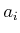to vary. More complicated joints can be modeled as a sequence of degenerate joints. For example, a spherical joint can be considered as a sequence of three zero-length revolute joints; the joints perform a roll, a pitch, and a yaw. Another option for more complicated joints is to abandon the DH representation and directly develop the homogeneous transformation matrix. This might be needed to preserve topological properties that become important in Chapter 4.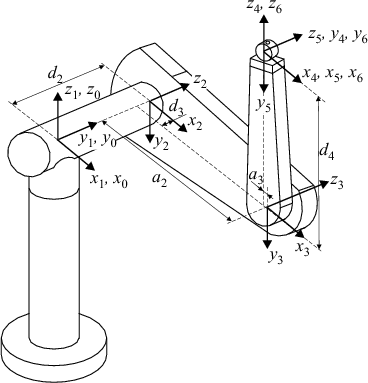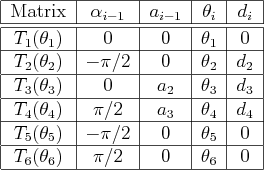Example 3..4 (Puma 560)   This example demonstrates the 3D chain kinematics on a classic robot manipulator, the PUMA 560, shown in Figure 3.16. The current parameterization here is based on [37,555]. The procedure is to determine appropriate body frames to represent each of the links. The first three links allow the hand (called an end-effector) to make large movements in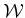, and the last three enable the hand to achieve a desired orientation. There are six degrees of freedom, each of which arises from a revolute joint. The body frames are shown in Figure 3.16, and the corresponding DH parameters are given in Figure 3.17. Each transformation matrixis a function of; hence, it is written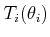. The other parameters are fixed for this example. Only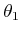,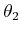,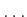,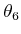are allowed to vary.

The parameters from Figure 3.17 may be substituted into the homogeneous transformation matrices to obtain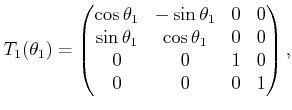(3.58)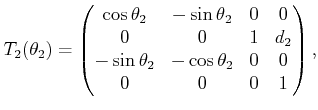(3.59)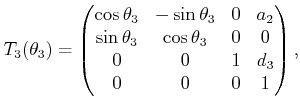(3.60)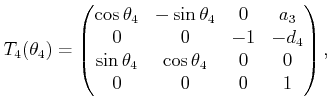(3.61)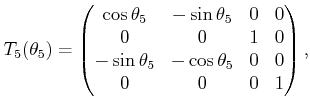(3.62)

and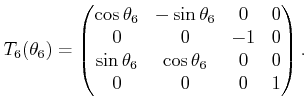(3.63)

A pointin the body frame of the last link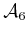appears inas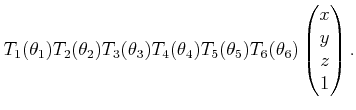(3.64)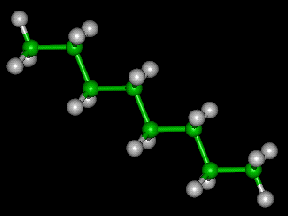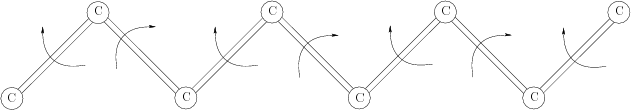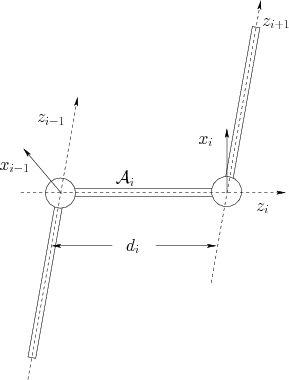Example 3..5 (Transforming Octane)   Figure 3.18 shows a ball-and-stick model of an octane molecule. Each ball'' is an atom, and each stick'' represents a bond between a pair of atoms. There is a linear chain of eight carbon atoms, and a bond exists between each consecutive pair of carbons in the chain. There are also numerous hydrogen atoms, but we will ignore them. Each bond between a pair of carbons is capable of twisting, as shown in Figure 3.19. Studying the configurations (called conformations) of molecules is an important part of computational biology. It is assumed that there are seven degrees of freedom, each of which arises from twisting a bond. The techniques from this section can be applied to represent these transformations.

Note that the bonds correspond exactly to the axes of rotation. This suggests that theaxes should be chosen to coincide with the bonds. Since consecutive bonds meet at atoms, there is no distance between them. From Figure 3.15c, observe that this makes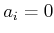for all. From Figure 3.15a, it can be seen that eachcorresponds to a bond length, the distance between consecutive carbon atoms. See Figure 3.20. This leaves two angular parameters,and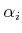. Since the only possible motion of the links is via rotation of the-axes, the angle between two consecutive axes, as shown in Figure 3.15d, must remain constant. In chemistry, this is referred to as the bond angle and is represented in the DH parameterization as. The remainingparameters are the variables that represent the degrees of freedom. However, looking at Figure 3.15b, observe that the example is degenerate because each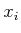-axis has no frame of reference because each. This does not, however, cause any problems. For visualization purposes, it may be helpful to replaceandby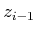and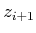, respectively. This way it is easy to see that as the bond for the-axis is twisted, the observed angle changes accordingly. Each bond is interpreted as a link,. The origin of eachmust be chosen to coincide with the intersection point of the- and-axes. Thus, most of the points inwill lie in the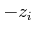direction; see Figure 3.20.

The next task is to write down the matrices. Attach a world frame to the first bond, with the second atom at the origin and the bond aligned with the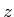-axis, in the negative direction; see Figure 3.20. To define, recall thatfrom (3.54) becauseis dropped. The parameter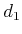represents the distance between the intersection points of the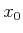- and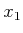-axes along the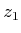axis. Since there is no-axis, there is freedom to choose; hence, let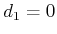to obtain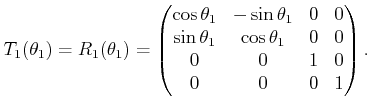(3.65)

The application ofto points incauses them to rotate around the-axis, which appears correct.

The matrices for the remaining six bonds are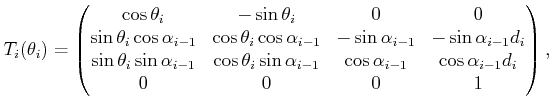(3.66)

for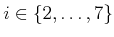. The position of any point,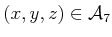, is given by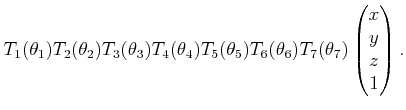(3.67)Steven M LaValle 2020-08-14